#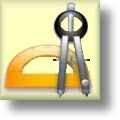Geometry Worksheets

## Area and Perimeter Worksheets

Here is a graphic preview for all of the Area and Perimeter Worksheets Sections. You can select different variables to customize these Area and Perimeter Worksheets for your needs. The Area and Perimeter Worksheets are randomly created and will never repeat so you have an endless supply of quality Area and Perimeter Worksheets to use in the classroom or at home. We have Area and Perimeter Worksheets for Triangles, Quadrilaterals, Regular Polygons, and a great Formula Worksheet for your use. Our Area and Perimeter Worksheets are free to download, easy to use, and very flexible.

Click here for a Detailed Description of all the Area and Perimeter Worksheets.

## Quick Link for All Area and Perimeter Worksheets

Click the image to be taken to that Area and Perimeter Worksheets.

##### Area and Perimeter FormulaWorksheets Page 1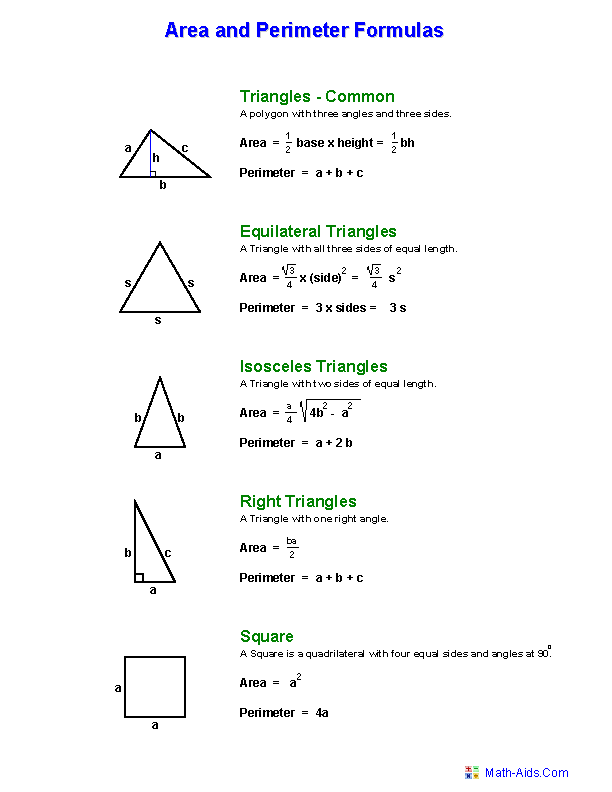##### Area and Perimeter FormulaWorksheets Page 2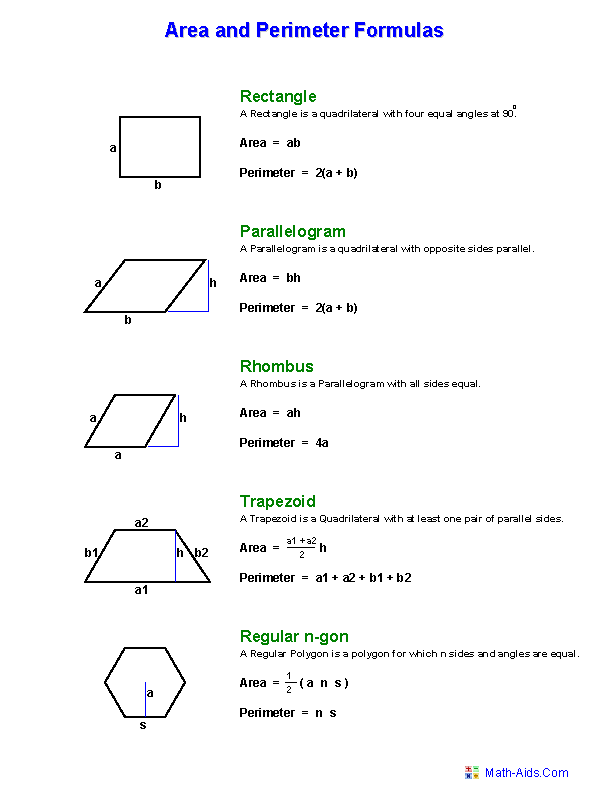##### Area and Perimeter ofTriangles Worksheets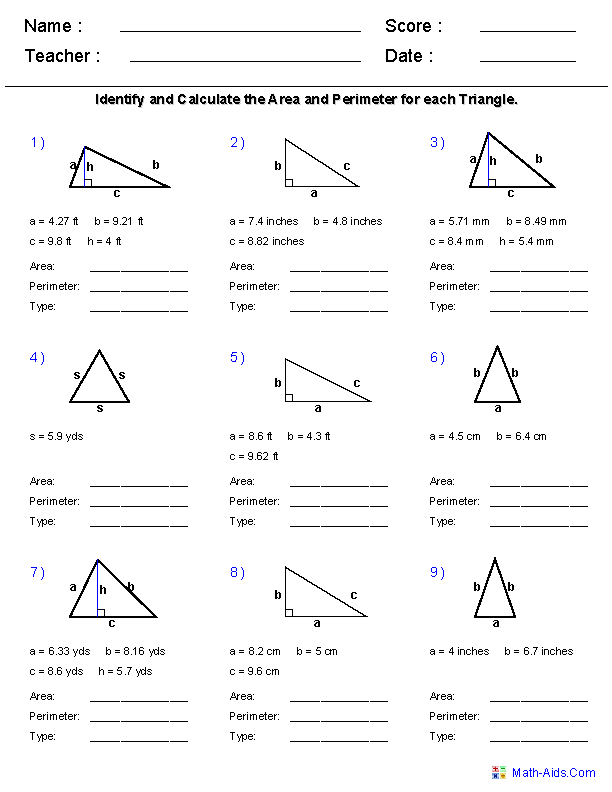##### Area and Perimeter ofQuadrilaterals Worksheets##### Area and Perimeter ofRegular Polygons Worksheets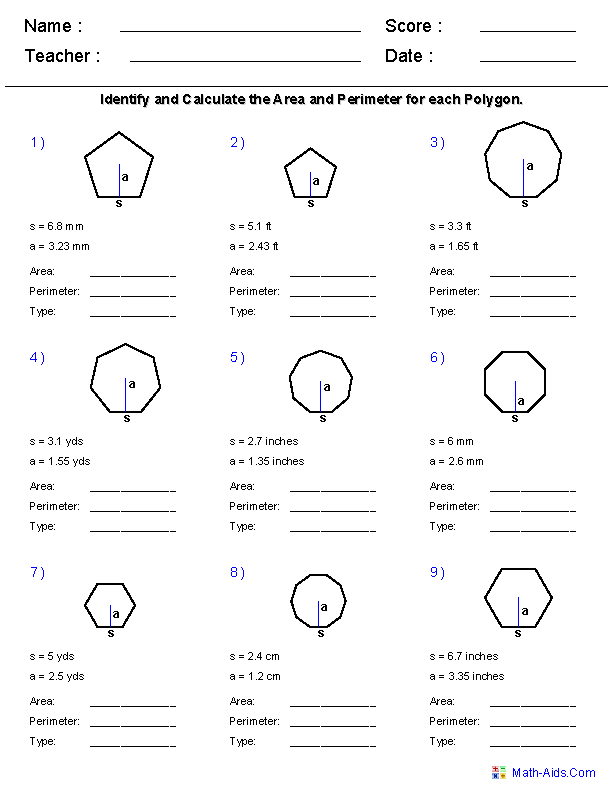##### Area and Perimeter UsingAll Polygons Worksheets##### Area of Compound ShapesAdding Regions Worksheets##### Area of Compound ShapesSubtracting Regions Worksheets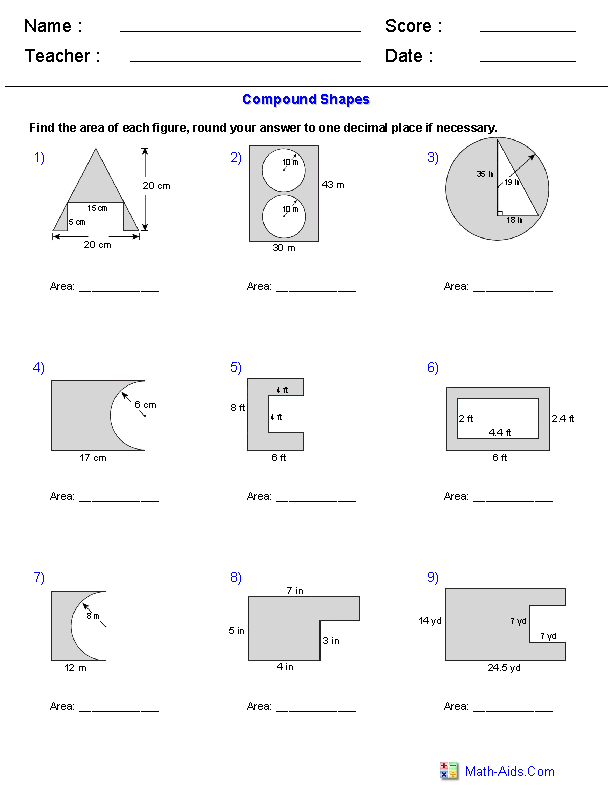##### Area of Compound ShapesAdding & Subtracting Regions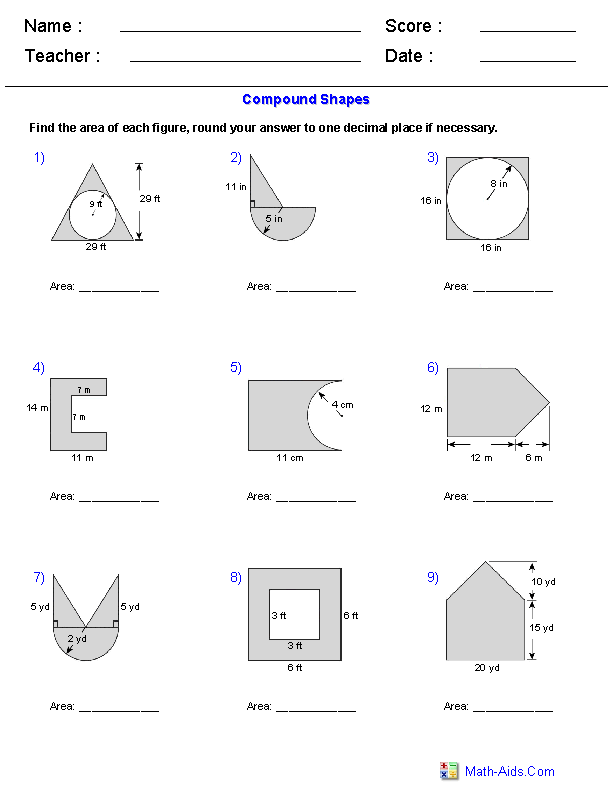Recommended Videos

## Detailed Description for All Area and Perimeter Worksheets

Area and Perimeter Formula Worksheets
These Area and Perimeter Worksheets will produce a formula reference worksheet which is a great handout for the students. The formulas produce are for the right triangle, common triangle, equilateral triangle, isosceles triangle, square, rectangle, parallelogram, rhombus, trapezoid, pentagon, hexagon, and octagon. These worksheet are a great resources for the 5th, 6th Grade, 7th Grade, and 8th Grade.

Area and Perimeter of Triangles Worksheets
These Area and Perimeter Worksheets will produce nine problems for solving the area and perimeter for right triangles, common triangles, equilateral triangles, and isosceles triangles. These worksheet are a great resources for the 5th, 6th Grade, 7th Grade, and 8th Grade.

Area and Perimeter of Quadrilaterals Worksheets
These Area and Perimeter Worksheets will produce nine problems for solving the area and perimeter for squares, rectangles, parallelograms, rhombuses, and trapezoids. These worksheet are a great resources for the 5th, 6th Grade, 7th Grade, and 8th Grade.

Area and Perimeter of Regular Polygons Worksheets
These Area and Perimeter Worksheets will produce nine problems for solving the area and perimeter for pentagons, hexagons, heptagons, octagons, nonagons, decagons, hendecagons, and dodecagons. These worksheet are a great resources for the 5th, 6th Grade, 7th Grade, and 8th Grade.

Area and Perimeter Using All Polygons Worksheets
These Area and Perimeter Worksheets will produce nine problems for solving the area and perimeter for right triangles, common triangles, equilateral triangles, isosceles triangles, squares, rectangles, parallelograms, rhombuses, trapezoids, pentagons, hexagons, heptagons, octagons, nonagons, decagons, hendecagons, and dodecagons. These worksheet are a great resources for the 5th, 6th Grade, 7th Grade, and 8th Grade.

Area of Compound Shapes - Adding Regions Worksheets
These Area Worksheets will produce problems for finding the area of compound shapes that are comprised of adding regions of simple figures. You can select the types of figures used and the units of measurement. These worksheet are a great resources for the 5th, 6th Grade, 7th Grade, and 8th Grade.

Area of Compound Shapes - Subtracting Regions Worksheets
These Area Worksheets will produce problems for finding the area of compound shapes that are comprised of subtracting regions of simple figures. You can select the types of figures used and the units of measurement. These worksheet are a great resources for the 5th, 6th Grade, 7th Grade, and 8th Grade.

Area of Compound Shapes - Adding & Subtracting Regions Worksheets
These Area Worksheets will produce problems for finding the area of compound shapes that are comprised of adding and subtracting regions of simple figures. You can select the types of figures used and the units of measurement. These worksheet are a great resources for the 5th, 6th Grade, 7th Grade, and 8th Grade.